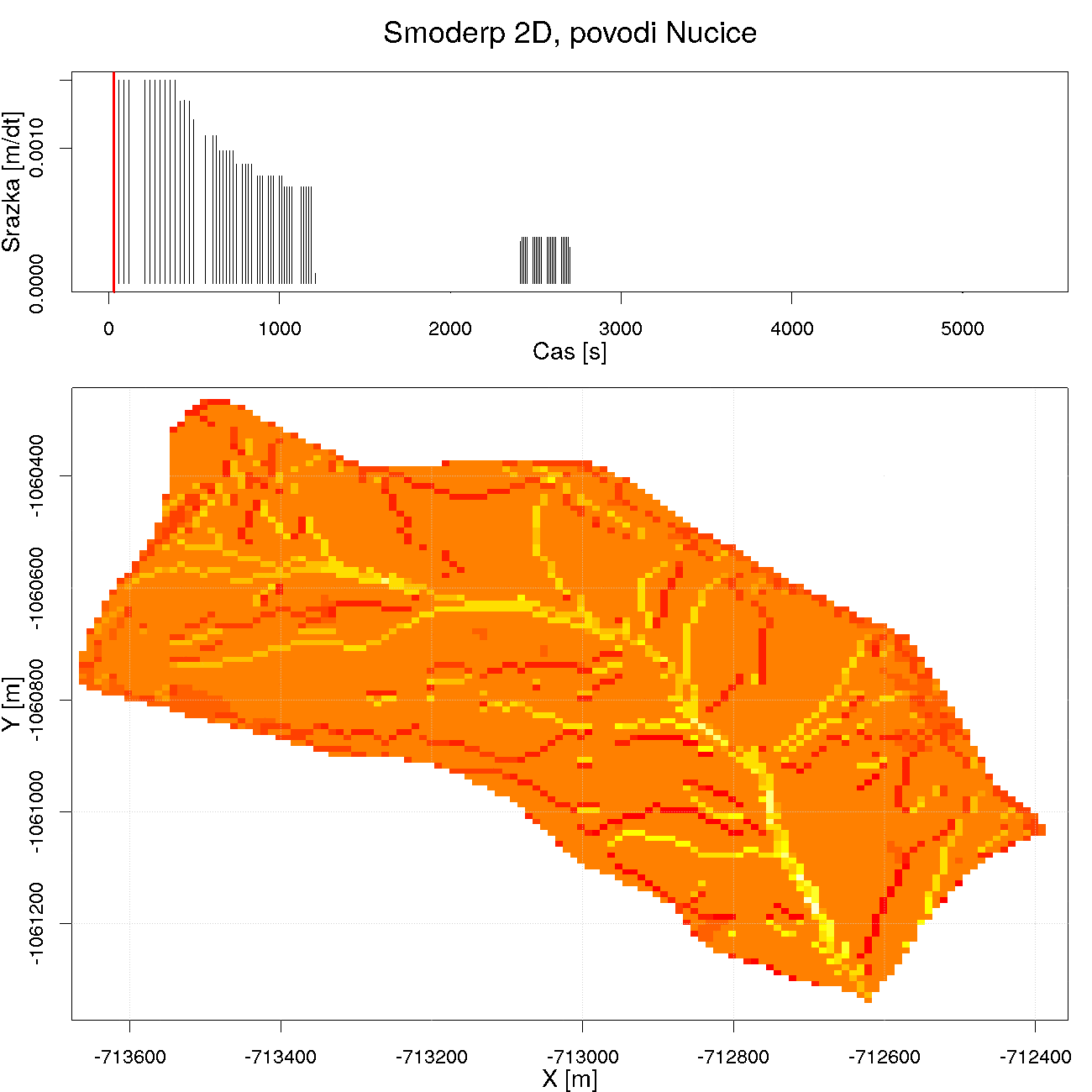CZ | EN

Návrhové srážky
(22.02.2023 v 18:01)

V rámci projektu "Předchozí nasycenost a návrhové srážkové intenzity jako faktory odtokové odezvy na malých povodích" byly aktualizovány data návrhových šestihodinových srážek. Detaily naleznete na portálu rain.fsv.cvut.cz

Our researchers in media
(4.08.2022 v 10:45)

## Interviews here

Calendar
(3.10.2023 v 10:47)

Krajina a voda (24.-25.10.2023)

GISday 2023 (15.11.2023)

# SMODERP 2D

The 2D model which is developed in last years is prepared as an extension to the widespread GIS software ArcGIS. The actual script to calculate the 2D model was elaborated in Python and used in ArcGIS standard tools.

Moreover, besides the mathematical calculation of surface runoff, the 2D model also includes a submodel implemented for runoff calculation in the rills

Rill runoff is an additional physic process which is implemented in the model. For each soil type, a critical value of the tangential stress and velocity was estimated. From this critical value, the critical height in each cell is calculated. In principle, this is a comparison of the current level and its critical value at each time interval. If the critical value is exceeded, the calculation enters the stage at which the rill starts to form. Dimensions of the rills are calculated from the volume of the water exceeding the critical value. Sheet surface runoff is then calculated using the critical value level instead of current height in the time step. Once the level has dropped below the critical height value, the calculation returns only in the calculation of surface runoff. The resulting rasters of rill flow and speed in the rill are stored in the user-selected directory along with vector shapefile of created rills. Calculation of the flow in the rill is based on the Manning equation.